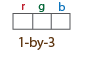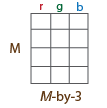Insert masks in image or video stream

Since R2020b

## Syntax

``RGB = insertObjectMask(I,BW)``
``RGB = insertObjectMask(I,maskstack)``
``RGB = insertObjectMask(___,Name=Value)``

## Description

example

````RGB = insertObjectMask(I,BW)` inserts a mask `BW` into the specified image `I` and returns the result as a truecolor image `RGB`.```

example

````RGB = insertObjectMask(I,maskstack)` inserts a set of masks `maskstack` into the specified image `I` and returns the result as a truecolor image `RGB`.```
````RGB = insertObjectMask(___,Name=Value)` specifies options using one or more name-value arguments in addition to any combination of arguments from previous syntaxes. For example, `(MaskColor="red")` sets the color of the mask to red.```

## Examples

collapse all

Read an image into the workspace.

`I = imread("visionteam1.jpg");`

`load("visionteam1Maskstack.mat")`

Insert the masks with white outlines into the image.

`RGB = insertObjectMask(I,maskstack,LineColor="white",LineWidth=2);`

Display the image with the masks inserted.

```figure imshow(RGB)```Read an image into the workspace.

`I = imread("visionteam1.jpg");`

`load("visionteam1Maskstack.mat");`

Insert the masks into the image and specify a unique color for each mask.

```numMasks = size(maskstack,3); RGB = insertObjectMask(I,maskstack,MaskColor=lines(numMasks));```

Display the image with the inserted masks.

```figure imshow(RGB)```## Input Arguments

collapse all

Input image, specified as a truecolor (RGB) image, m-by-n-by-3 array, or a grayscale image, m-by-n array.

Data Types: `single` | `double` | `int16` | `uint8` | `uint16`

Input mask image, specified as an m-by-n logical matrix.

Data Types: `logical`

Stack of mask images, specified as an m-by-n-by-P logical array, where P is the total number of masks in the stack.

`maskstack` has the same width and height as `I`.

Data Types: `logical`

### Name-Value Arguments

Specify optional pairs of arguments as `Name1=Value1,...,NameN=ValueN`, where `Name` is the argument name and `Value` is the corresponding value. Name-value arguments must appear after other arguments, but the order of the pairs does not matter.

Before R2021a, use commas to separate each name and value, and enclose `Name` in quotes.

Example: `LineColor="white"` set the inserted line color to white.

Mask color, specified as a character vector, cell array of character vectors, vector, or M-by-3 matrix of RGB values.

An RGB triplet is a three-element row vector whose elements specify the intensities of the red, green, and blue components of the color. The intensities must be in the range `[0,1]`, for example, ```[0.4 0.6 0.7]```. When using a data type of `uint8`, which is defined within the range of `[0,255]`, conversions are necessary to ensure that the expected range is met. For example, to specify the previous vector, you must convert it by using `uint8(255*[0.4 0.6 0.7])`.

You can specify a different color for each mask or one color for all masks. To specify one color for all masks, set `MaskColor` to a color string or an [R G B] vector.

SpecificationFormatExample
Specify one color for all shapes (or markers)

Short color name or color name

`"r"`

`"red"`

RGB triplet

`[1 0 0]`Specify a color for each shape (or marker)

Vector of color names

`["red","yellow","blue"]`

Three-column matrix of RGB triplets

```[1 0 0 0 1 1 1 0 1 1 1 1]```Supported colors are listed in the table.

Color NameShort NameRGB TripletAppearance
`"red"``"r"``[1 0 0]``"green"``"g"``[0 1 0]``"blue"``"b"``[0 0 1]``"cyan"` `"c"``[0 1 1]``"magenta"``"m"``[1 0 1]``"yellow"``"y"``[1 1 0]``"black"``"k"``[0 0 0]``"white"``"w"``[1 1 1]`Color values must be specified in the range of the data type support that you use to specify them. For example, if you specify an input of `double` datatype, then values must be in the range `[0,1]`. If you specify an input of `uint8` datatype, then values must be in the range `[0 255]`. For example, `[0,0,1]` and `uint8([0,0,255])` are two formats for the same color blue.

Data Types: `logical` | `uint8` | `uint16` | `int16` | `double` | `single`

Opacity of mask, specified as a scalar value in the range [0 1]. The value `1` makes the mask completely opaque and the value `0` makes the mask completely transparent.

Data Types: `single` | `double` | `int8` | `int16` | `int32` | `int64` | `uint8` | `uint16` | `uint32` | `uint64`

Color of mask borders, specified as one of these values:

• `"auto"``LineColor` uses the same value or values as `MaskColor`.

• P-by-3 matrix of RGB triplets, where P is the total number of masks

• P-element vector of MATLAB® ColorSpec names

• 1-by-3 RGB triplet or scalar MATLAB ColorSpec name, specifying the color to use for all masks.

For a description of RGB color and how to specify it, see `MaskColor`.

Data Types: `single` | `double` | `int16` | `uint8` | `uint16` | `logical` | `char` | `string`

Opacity of the mask borders, specified as a scalar value in the range of [0 1].

Data Types: `single` | `double` | `int8` | `int16` | `int32` | `int64` | `uint8` | `uint16` | `uint32` | `uint64`

Width of mask borders, specified as a positive scalar in pixels.

Data Types: `single` | `double` | `int8` | `int16` | `int32` | `int64` | `uint8` | `uint16` | `uint32` | `uint64`

## Output Arguments

collapse all

Output image, returned as a truecolor image of same datatype as the input image, with the same m-by-n dimensions as `I`.

## Tips

• When masks overlap and the same pixel is in more than one mask, `maskstack(:,:,i)` takes precedence over `maskstack(:,:,j)`, where `i` < `j`.

• For better performance, set `LineOpacity` to `0` to disable drawing of the edges.

## Version History

Introduced in R2020b

expand all